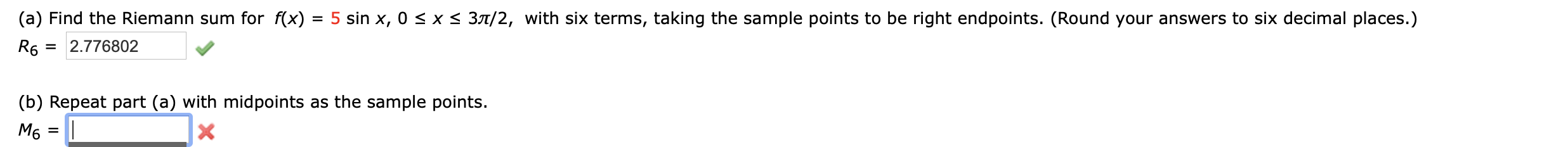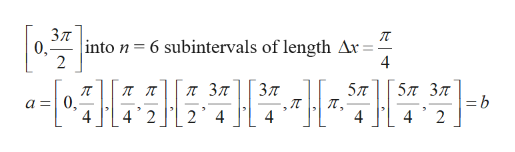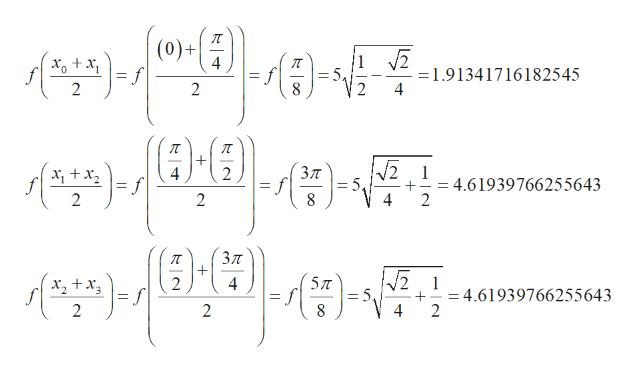# x s 3T/2, with six terms, taking the sample points to be right endpoints. (Round your answers to six decimal places.)(a) Find the Riemann sum for f(x)= 5 sin x, 0R62.776802(b) Repeat part (a) with midpoints as the sample points.M6

Question
8 viewshelp_outlineImage Transcriptionclosex s 3T/2, with six terms, taking the sample points to be right endpoints. (Round your answers to six decimal places.) (a) Find the Riemann sum for f(x) = 5 sin x, 0 R6 2.776802 (b) Repeat part (a) with midpoints as the sample points. M6 fullscreen
check_circle

Step 1

Repeate part (a)

Riemann sum for

Step 2

Divide the intervalhelp_outlineImage TranscriptioncloseЗл into n = 6 subintervals of length Ax = 0, 2 л Зл Зл 5л 5π 3π =b 2 a =0. 4 4 2 24 4 4 fullscreen
Step 3

Now, we just evaluate the func...help_outlineImage Transcriptionclose(0)+ 4 +x =f 2 f 8 =1.91341716182545 4 2 2 1 = 5. Зл 2 f 2 4.61939766255643 2 2 Зл /2 1 5TT 2 = f x,+x f 2 = 4.61939766255643 2 f = 5. 8 4 2 fullscreen

### Want to see the full answer?

See Solution

#### Want to see this answer and more?

Solutions are written by subject experts who are available 24/7. Questions are typically answered within 1 hour.*

See Solution
*Response times may vary by subject and question.
Tagged in

### Calculus